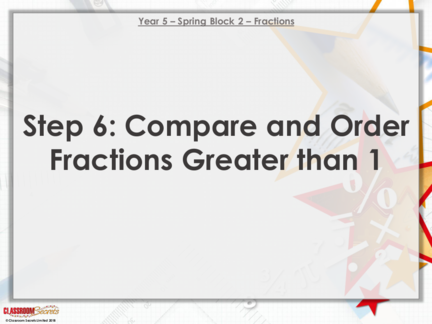# Comparing and Ordering Fractions WorksheetsContributed by:Comparing and ordering fractions worksheet. A fraction represents a part of a whole or, more generally, any number of equal parts. A fraction describes how many parts of a certain size there are, for example, one-half, eight-fifths, three-quarters.
1. Year 5 – Spring Block 2 – Fractions
Step 6: Compare and Order
Fractions Greater than 1
2. Introduction
Circle the fractions which are greater than 1.
12 11 12
6 10 15
5 13
5 12
7 8 20
14 9 19
How do you know they are bigger than 1?
What do we call these types of fractions?
3. Introduction
Circle the fractions which are greater than 1.
12 11 12
6 10 15
5 13
5 12
7 8 20
14 9 19
4. Varied Fluency 1
Using the representations below, complete the statement.
>
The fraction of
How many full
the last bar.
bars?